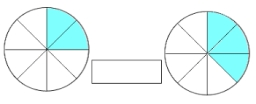# The product 4

The product of two simple fraction is 12/35. If one of the fractions is 3/7, what is the other one?Did you find an error or inaccuracy? Feel free to write us. Thank you!

Showing 1 comment:Vinco
Q: 5+1-2=?
A: 4Tips to related online calculators
Need help to calculate sum, simplify or multiply fractions? Try our fraction calculator.
Do you have a linear equation or system of equations and looking for its solution? Or do you have a quadratic equation?